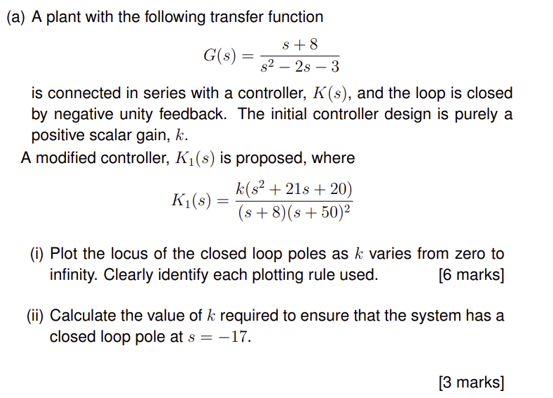Home / Expert Answers / Mechanical Engineering / a-a-plant-with-the-following-transfer-function-g-s-frac-s-8-s-2-2-s-pa657

# (Solved): (a) A plant with the following transfer function $G(s)=\frac{s+8}{s^{2}-2 s ...??????? (a) A plant with the following transfer function \[ G(s)=\frac{s+8}{s^{2}-2 s-3}$ is connected in series with a controller, $$K(s)$$, and the loop is closed by negative unity feedback. The initial controller design is purely a positive scalar gain, $$k$$. A modified controller, $$K_{1}(s)$$ is proposed, where $K_{1}(s)=\frac{k\left(s^{2}+21 s+20\right)}{(s+8)(s+50)^{2}}$ (i) Plot the locus of the closed loop poles as $$k$$ varies from zero to infinity. Clearly identify each plotting rule used. [6 marks] (ii) Calculate the value of $$k$$ required to ensure that the system has a closed loop pole at $$s=-17$$.

We have an Answer from Expert